Finding Area of Quadrilaterals using Herons Formula - Herons Formula, Class 9, Mathema

# Finding Area of Quadrilaterals using Herons Formula - Herons Formula, Class 9, Mathema - Extra Documents & Tests for Class 9

APPLICATIONS OF HERON'S FORMULA IN FINDING AREA OF A QUADRILATERAL

Heron's formula can be applied to find the area of a quadrilateral by dividing the quadrilateral into two triangular parts. If we join any of the two diagonals of the quadrilateral, then we get two triangles. Area of each triangle is calculated and the sum of two areas is the area of the quadrilateral.

1. RHOMBUS : A quadrilateral whose all the sides are equal and opposite sides are parallel. The diagonals of a rhombus bisect each other at right angle.
In figure, ABCD is a rhombus with side a and AC and BD are the two diagonals and AC = d1, BD = d2, then

Perimeter = 4a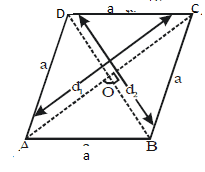Area = Area of ΔABD + Area of ΔCDB

= 1/2. BD . OA + 1/2 . BD . OC
= 1/2.BD[OA + OC] = 1/2.BD . AC = 1/2. × d1 × dor 1/2 . × product of diagonals.

2. TRAPEZIUM : A quadrilateral which has one pair of opposite sides parallel.

In figure, ABCD is a trapezium with AB || CD,

AB = b, BC = c, CD = a, DA = d and the height of the trapezium ABCD is h, then

Perimeter = a + b + c + d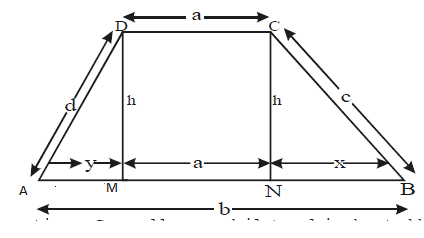Area = Area of ΔAMD + Area of rectangle MNCD + Area of  ΔCNB
= 1/2. y . h + a . h + 1/2 . x . h
= 1/2. h (x + y) + ah = 1/2. h [x + y + 2a]
= 1/2. h [(x + y + a) + a] = 1/2. h [b + a]
= 1/2. (a + b) h = 1/2. (Sum of the parallel sides) × Distance between them.

3. QUADRILATERAL : A figure, which has four sides and four vertices. Generally a quadrilateral is denoted bythe symbol 'rectangle'.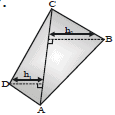In figure, ABCD is a quadrilateral in which AC is a diagonal and AC divides quadrilateral ABCD in two triangle ADC and ABC. Also, h1 and h2 are the altitudes of the triangle ADC and ABC respectively.
∴ Area = Area of ΔADC + Area of ΔABC

=1/2 . AC . h1 + 1/2. AC . h2 = 1/2. AC (h1 + h2)
=  1/2 . × One diagonal × (Sum of heights of those triangles whose base is former diagonal)

Ex. Find the area of the quadrilateral ABCD, in which AB = 7 cm, BC = 6 cm, CD= 12 cm, DA = 15 cm and AC = 9 cm.

Sol.The diagonal AC divides the quadrilateral ABCD into two triangles ABC and ACD.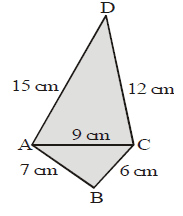Area of quad. ABCD = Area of AABC + Area of ΔACD, For ΔABC, we have

Semiperuneter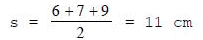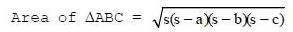⇒ A1 = Area of ΔABC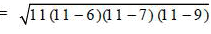⇒ At = Area of ΔABC =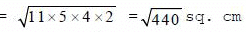⇒ At = Area of ΔABC = 20.98 cm2
For ΔACD, we have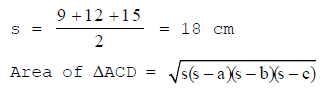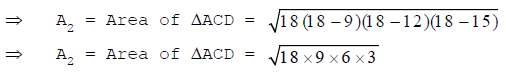⇒ A2 = Area of ΔACD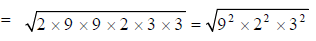= 9 × 2 × 3 = 54 cm2
Hence, Area of quad. ABCD = A1 + A2 = (20.98 + 54) cm2 = 74.98 cm2

Ex. Prove that the area of the quadrilateral ABCD is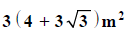if AB = 5 m, BC = 5 m, CD = 6 m,AD = 6 m, and diagonal AC = 6 m.

Sol. Diagonal AC divides the quadrilateral ABCD into two triangles ΔACD and ΔABC.
For ΔACD, side are 6m, 6 m and 6 m.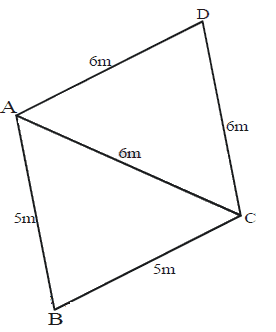Semiperimeter,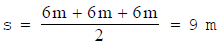∴ Area of ΔACD =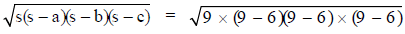m2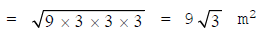For ΔABC, side are 5 m, 5 m and 6 m.
Semiperimeter,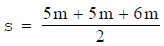= 8m

Area of ΔABC =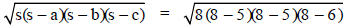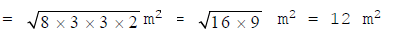Thus, the area of the quadrilateral ABCD =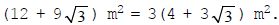Ex. In fig. ABCD is a field in the form of a quadrilateral whose sides are indicated in the figure. lf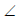DAB = 90°, find the area of the field.

Sol. Clearly, ΔDAB is a right-angled triangle. Therefore,
DB2 = DA2 + AB2 [Using Pythagoras Theorem]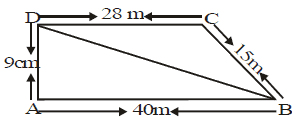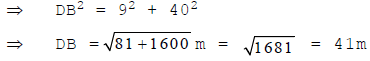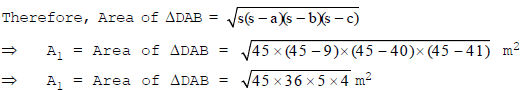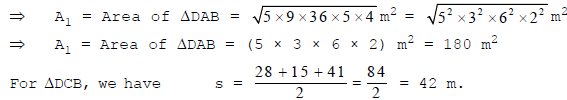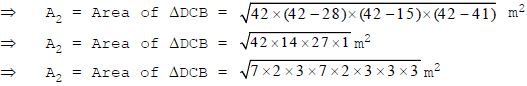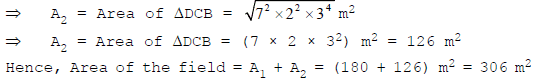Ex. A rhombus has perimeter 100 m and one of its diagonal is 40 m. Find the area of the rhombus.

Sol. ABCD is the rhombus having perimeter = 100 m and AC = 40 m.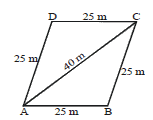Now, we have AB = BC = CD = AD =100/4 m = 25 m

We know that, ar (ΔABC) = ar (ΔADC)

Sides of ΔABC are 25 m, 25 m and 40 m.
Semi perimeter of ΔABC (s)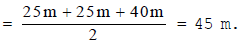The area of ΔABC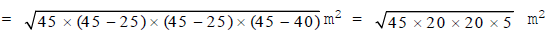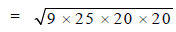= 3 × 5 × 20 m= 300 m2
Also, we havearea of ΔADC = 300 m2.
Hence, the area of the rhombus ABCD = ar (ΔABC) + ar (ΔADC) = 300 m2 + 300 m2 = 600 m2.

 THINGS TO REMEMBER1. Area of a triangle, whose base and height are known, is calculated by using the formula:Area of triangle =1/2× Base × Height2. Area of a right angled triangle, whose base is b and perpendicular is p, is calculated by using the formula:Area of triangle = 1/2× bp3. Area of an equilateral triangle, each of whose side is a, is calculated by using the formula:Area of triangle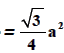4. Area of an isosceles triangle, each of whose equal sides is a and the unequal side is b, is calculated by using the formula:Area of triangle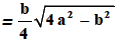5. Area of a triangle with its sides as a,b, and c is calculated by using Heron's formula :Area of triangle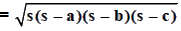where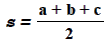The document Finding Area of Quadrilaterals using Herons Formula - Herons Formula, Class 9, Mathema | Extra Documents & Tests for Class 9 is a part of the Class 9 Course Extra Documents & Tests for Class 9.
All you need of Class 9 at this link: Class 9

## Extra Documents & Tests for Class 9

1 videos|228 docs|21 tests

## FAQs on Finding Area of Quadrilaterals using Herons Formula - Herons Formula, Class 9, Mathema - Extra Documents & Tests for Class 9

 1. What is Heron's formula?Ans. Heron's formula is a mathematical formula used to find the area of a triangle when all three sides of the triangle are known. It is also known as Hero's formula.
 2. Can Heron's formula be used to find the area of a quadrilateral?Ans. Yes, Heron's formula can be used to find the area of a quadrilateral when the four sides and a diagonal of the quadrilateral are known. The quadrilateral can be split into two triangles, and the area of each triangle can be calculated using Heron's formula.
 3. How do you split a quadrilateral into two triangles to use Heron's formula?Ans. To split a quadrilateral into two triangles, draw a diagonal from one vertex to another vertex. This will divide the quadrilateral into two triangles. Then, the area of each triangle can be calculated using Heron's formula.
 4. What is the advantage of using Heron's formula to find the area of a quadrilateral?Ans. The advantage of using Heron's formula to find the area of a quadrilateral is that it provides an accurate result without the need for advanced techniques. The formula is easy to use and can be applied to any quadrilateral with known sides and a diagonal.
 5. Is it possible to use Heron's formula to find the area of a quadrilateral when only the four sides are known?Ans. No, it is not possible to use Heron's formula to find the area of a quadrilateral when only the four sides are known. To use Heron's formula, a diagonal of the quadrilateral must also be known.

## Extra Documents & Tests for Class 9

1 videos|228 docs|21 testsExplore Courses for Class 9 examSignup to see your scores go up within 7 days! Learn & Practice with 1000+ FREE Notes, Videos & Tests.
10M+ students study on EduRev
Track your progress, build streaks, highlight & save important lessons and more!
Related Searches

,

,

,

,

,

,

,

,

,

,

,

,

,

,

,

,

,

,

,

,

,

,

,

,

,

,

,

;